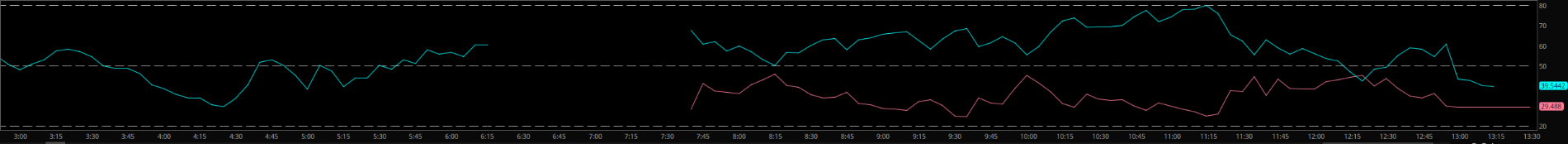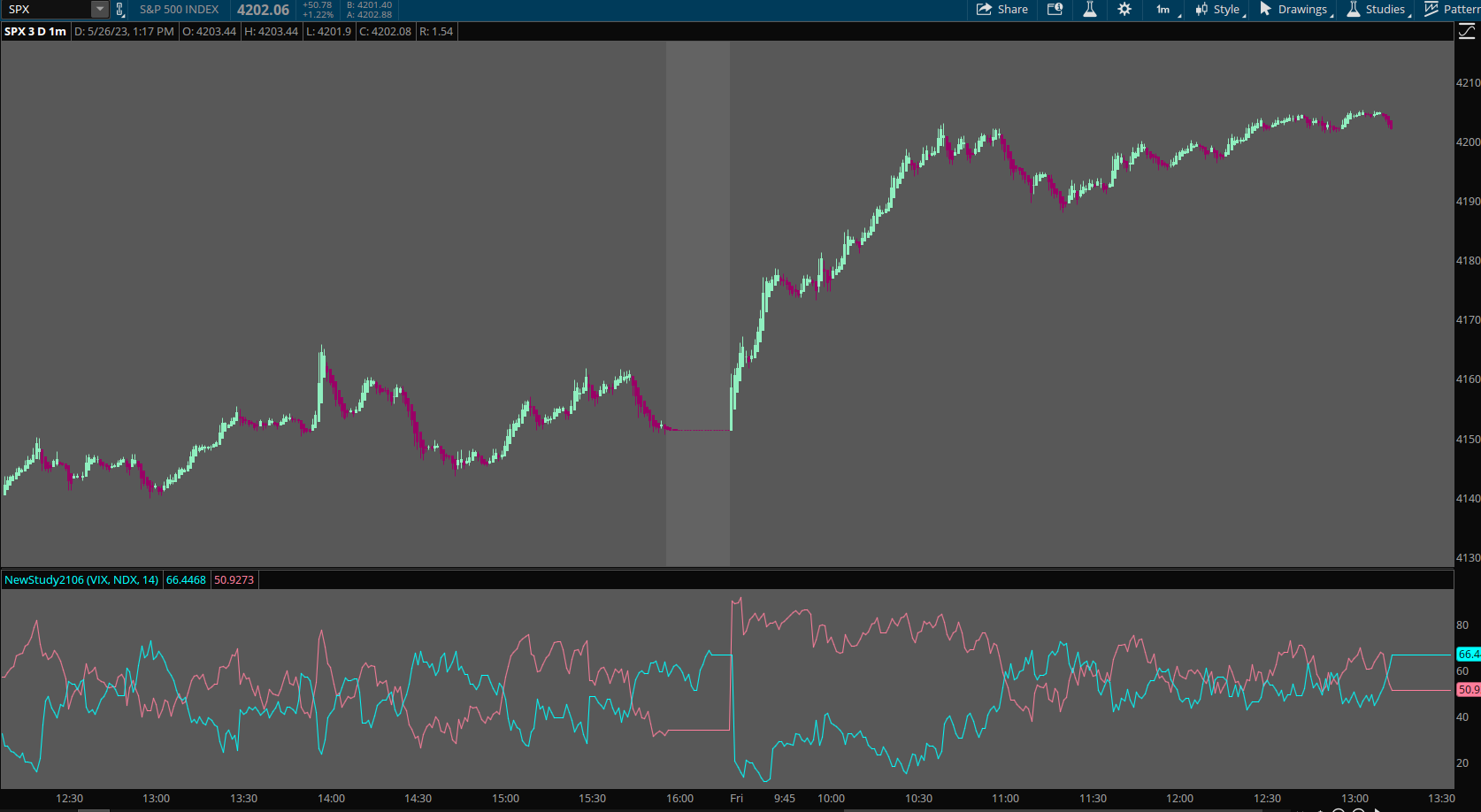# Data missing or script is wrong?

#### makc52

##### New member
Trying to PLOT RSI of VIX and NDX on the SPX chart, using the Charts time aggregate.

Code:
``````declare lower;

input security_num1 = "VIX";
input security_num2 = "NDX";

def vix = close(security_num1);
def src = close(security_num2);

input rsiLength = 14;

plot vixRSI = reference RSI("price" = vix, "length" = rsiLength);
plot nqRSI = reference RSI("price" = src, "length" = rsiLength);``````

Plotting the above seems to be cut off between 6:15 and 7:35. Any idea what is missing, is it data issue? Thank you!Solution
that's the thing, it's regular hours and it's for VIX and NDX so has to be something else

The use of a recursive (using prior value) helps when data is missing.

Code:
``````declare lower;

input security_num1 = "VIX";
input security_num2 = "NDX";

def vix = if isnan(close(security_num1)) then vix else close(security_num1);
def src = if isnan(close(security_num2)) then src else close(security_num2);
input rsiLength = 14;

plot vixRSI = reference RSI("price" = vix, "length" = rsiLength);
plot nqRSI = reference RSI("price" = src, "length" = rsiLength);``````#### Joshua

##### Well-known member
VIP
Its most likely because one or more of the symbols does not print during the same extended hours periods as the others. Should be fine if you use regular market hours.

#### makc52

##### New member
Its most likely because one or more of the symbols does not print during the same extended hours periods as the others. Should be fine if you use regular market hours.
that's the thing, it's regular hours and it's for VIX and NDX so has to be something else

Last edited:

#### SleepyZ

##### Well-known member
VIP
that's the thing, it's regular hours and it's for VIX and NDX so has to be something else

The use of a recursive (using prior value) helps when data is missing.

Code:
``````declare lower;

input security_num1 = "VIX";
input security_num2 = "NDX";

def vix = if isnan(close(security_num1)) then vix else close(security_num1);
def src = if isnan(close(security_num2)) then src else close(security_num2);
input rsiLength = 14;

plot vixRSI = reference RSI("price" = vix, "length" = rsiLength);
plot nqRSI = reference RSI("price" = src, "length" = rsiLength);``````•halcyonguy

### Not the exact question you're looking for?

87k+ Posts
337 Online## The Market Trading Game Changer

Join 2,500+ subscribers inside the useThinkScript VIP Membership Club
• Exclusive indicators
• Proven strategies & setups
• Private Discord community
• Exclusive members-only content
• 1 full year of unlimited support

What is useThinkScript?

useThinkScript is the #1 community of stock market investors using indicators and other tools to power their trading strategies. Traders of all skill levels use our forums to learn about scripting and indicators, help each other, and discover new ways to gain an edge in the markets.

How do I get started?

We get it. Our forum can be intimidating, if not overwhelming. With thousands of topics, tens of thousands of posts, our community has created an incredibly deep knowledge base for stock traders. No one can ever exhaust every resource provided on our site.

If you are new, or just looking for guidance, here are some helpful links to get you started.

What are the benefits of VIP Membership?